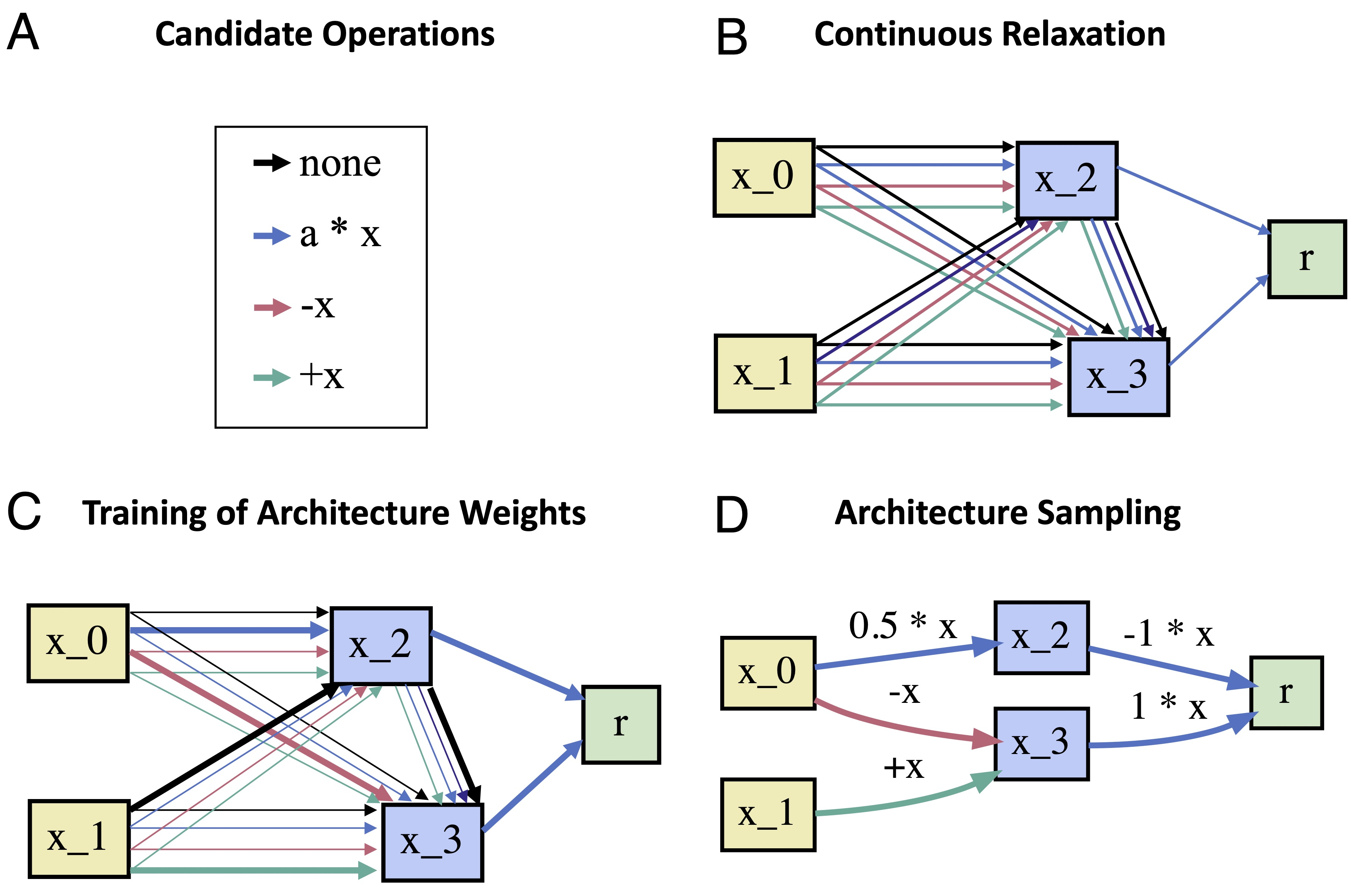# How It Works

Regular DARTS treats the architecture of a neural network as a directed acyclic computation graph (DAG), containing $$N$$ nodes in sequential order.Each node $$x_i$$ corresponds to a latent representation of the input space. Each directed edge $$e_{i, j}$$ is associated with some operation $$o_{i,j}$$ that transforms the representation of the preceding node $$i$$, and feeds it to node $$j$$. Each intermediate node is computed by integrating over its transformed predecessors:

$x_j = \sum_{i<j} o_{i,j} \left( x_{i} \right).$

Every output node is computed by linearly combining all intermediate nodes projecting to it. The goal of DARTS is to identify all operations $$o_{i,j}$$ of the DAG. Following Liu et al. (2019), we define {$$\mathscr{O} = \{o^1_{i,j}, o^2_{i,j}, \dots, o^M_{i,j}\}$$} to be the set of $$M$$ candidate operations associated with edge $$e_{i, j}$$ where every operation $$o^m_{i,j}(x_i)$$ corresponds to some function applied to $$x_{i}$$ (e.g. linear, exponential or logistic). DARTS relaxes the problem of searching over candidate operations by formulating the transformation associated with an edge as a mixture of all possible operations in $$\mathscr{O}$$ (cf. Figure A-B):

$\bar{o}_{i,j}(x) = \sum_{o \in \mathscr{O}} \frac{\textrm{exp}({\alpha^o_{i,j}})}{\sum_{o' \in \mathscr{O}} \textrm{exp}({\alpha^{o'}_{i,j}})} \cdot o_{i,j}(x).$

where each operation is weighted by the softmax transformation of its architectural weight $$\alpha^o_{i,j}$$. Every edge $$e_{i, j}$$ is assigned a weight vector $$\alpha_{i,j}$$ of dimension $$M$$, containing the weights of all possible candidate operations for that edge. The set of all architecture weight vectors $$\alpha = \{\alpha_{i,j}\}$$ determines the architecture of the model. Thus, searching the architecture amounts to identifying $$\alpha$$. The key contribution of DARTS is that searching $$\alpha$$ becomes amenable to gradient descent after relaxing the search space to become continuous. However, minimizing the loss function of the model $$\mathscr{L}(w,\alpha)$$ requires finding both $$\alpha^*$$ and $$w^*$$---the parameters of the computation graph.\footnote{This includes the parameters of each candidate operation $$o^m_{i,j}$$.} Liu et al. (2019) propose to learn $$\alpha$$ and $$w$$ simultaneously using bi-level optimization:

$\min_\alpha \mathscr{L}_{\textrm{val}}\left(w^*(\alpha),\alpha\right) \\ \textrm{s.t. } w^*(\alpha) = \underset{w}{\operatorname{argmin}} \mathscr{L}_{\textrm{train}}(w, \alpha).$

That is, one can obtain $$\alpha^*$$ through gradient descent, by iterating through the following steps:

• Obtain the optimal set of weights $$w^*$$ for the current architecture $$\alpha$$ by minimizing the training loss $$\mathscr{L}_{\textrm{train}}(w, \alpha)$$.
• Update the architecture $$\alpha$$ (cf. Figure C) by following the gradient of the validation loss $$\nabla \mathscr{L}_{\textrm{val}}\left(w^*,\alpha\right)$$.

Once $$\alpha^*$$ is found, one can obtain the final architecture by replacing $$\bar{o}_{i,j}$$ with the operation that has the highest architectural weight, i.e. $$o_{i,j}\leftarrow \textrm{argmax}_o \alpha^{*o}_{i,j}$$ (Figure D).

Liu, H., Simonyan, K., & Yang, Y. (2018). Darts: Differentiable architecture search. In International Conference on Learning Representations. arXiv: https://arxiv.org/abs/1806.09055